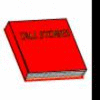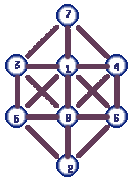You may also likeMonth Mania

Can you design a new shape for the twenty-eight squares and arrange the numbers in a logical way? What patterns do you notice?Neighbours

In a square in which the houses are evenly spaced, numbers 3 and 10 are opposite each other. What is the smallest and what is the largest possible number of houses in the square?Page Numbers

Exactly 195 digits have been used to number the pages in a book. How many pages does the book have?

1 to 8

Age 7 to 11Challenge Level

This solution was sent in by someone who only gave us their initial "S":1and 8 have only 2 and 7 next to them so I put them in the middle then I put 2 and 7 in the furthest away from their consecutive numbers. Then the next 2; 3 and 6 next to the numbers jut 2 away from them. the other 2 are easy 5 at the bottem and 4 at the top.

And here is an interesting way of approaching the problem sent in by Timothy. Notice that Timothy has turned the diagram on its side:

The solution to the above is:
6     4

2     8     1     7

5     3

How I did this was I numbered the circles 1 to 8 like this :

4     3

5     8     7     2

6     1

The way that I learned to do this puzzle is for a start put the numbers 1 and 8 in the middle circles. I then started by looking at the circle numbers that were not together, then I started making lists of the circle numbers that were not together, then I made larger lists of the circle numbers, and then each list taught me another step until I had all the pieces of information I needed. then I made the correct list which was:

7,5,1,3,6,4,2,8
1,2,3,4,5,6,7,8

The top row are the circle numbers, and the bottom row are the numbers which go into the circles.